# Honors Geometry Lateral Area Surface Area and Volume

• Slides: 29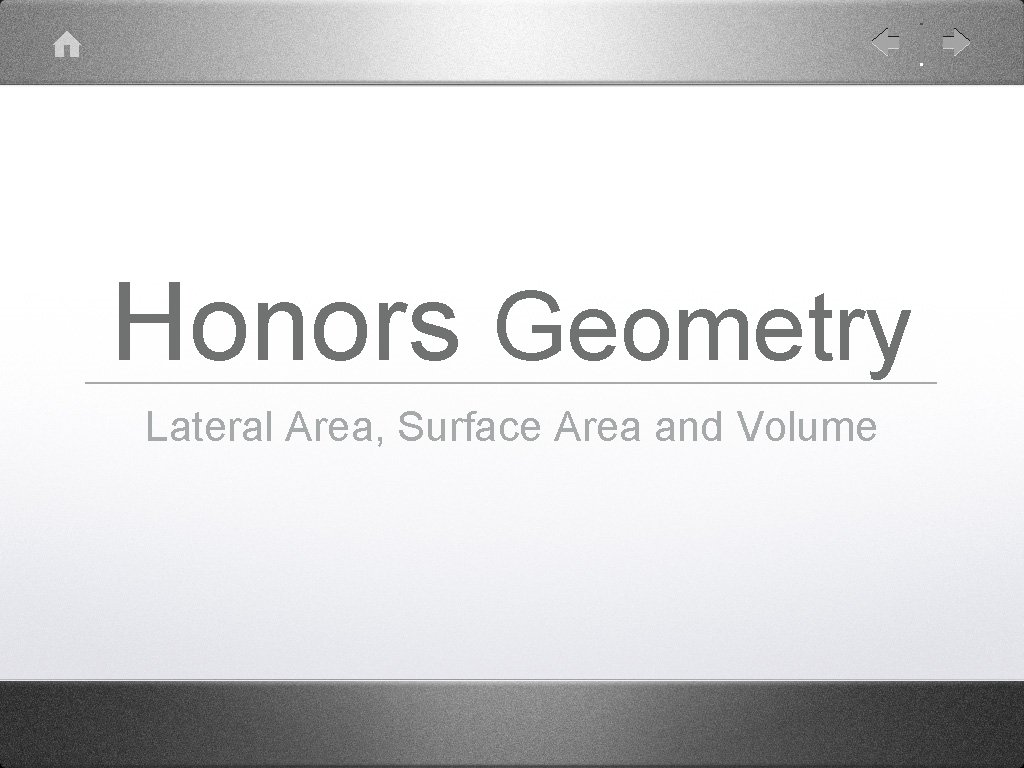Honors Geometry Lateral Area, Surface Area and Volume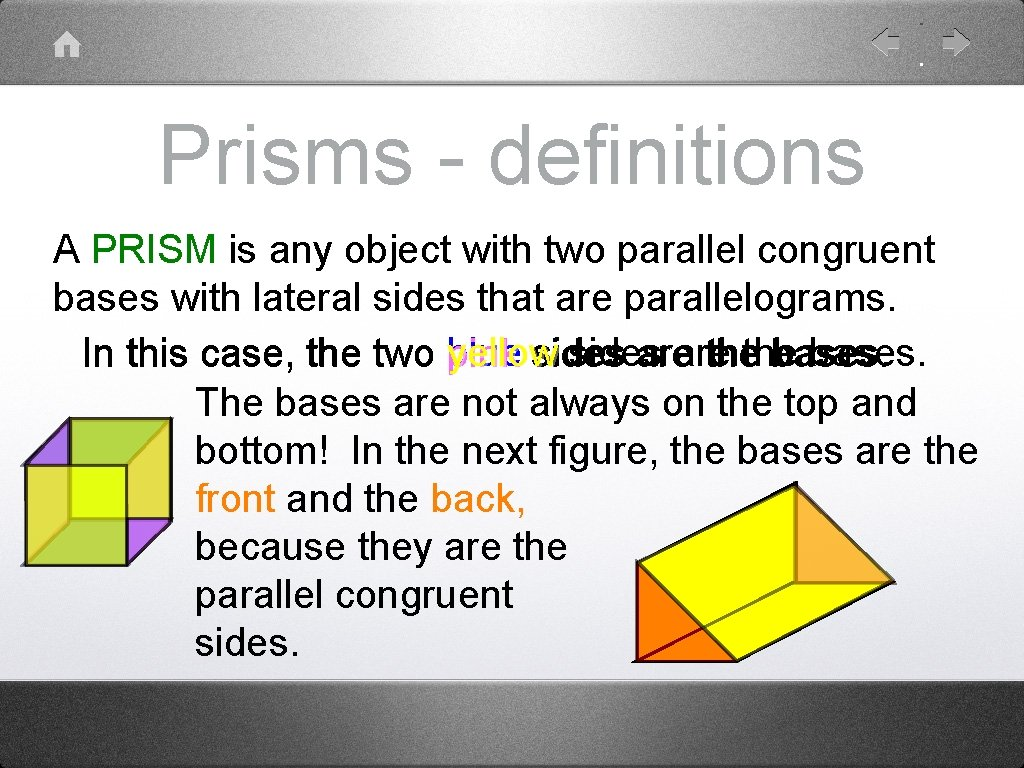Prisms - definitions A PRISM is any object with two parallel congruent bases with lateral sides that are parallelograms. In this case, the two blue yellow sides bases. pink sides areare thethe bases. The bases are not always on the top and bottom! In the next figure, the bases are the front and the back, because they are the parallel congruent sides.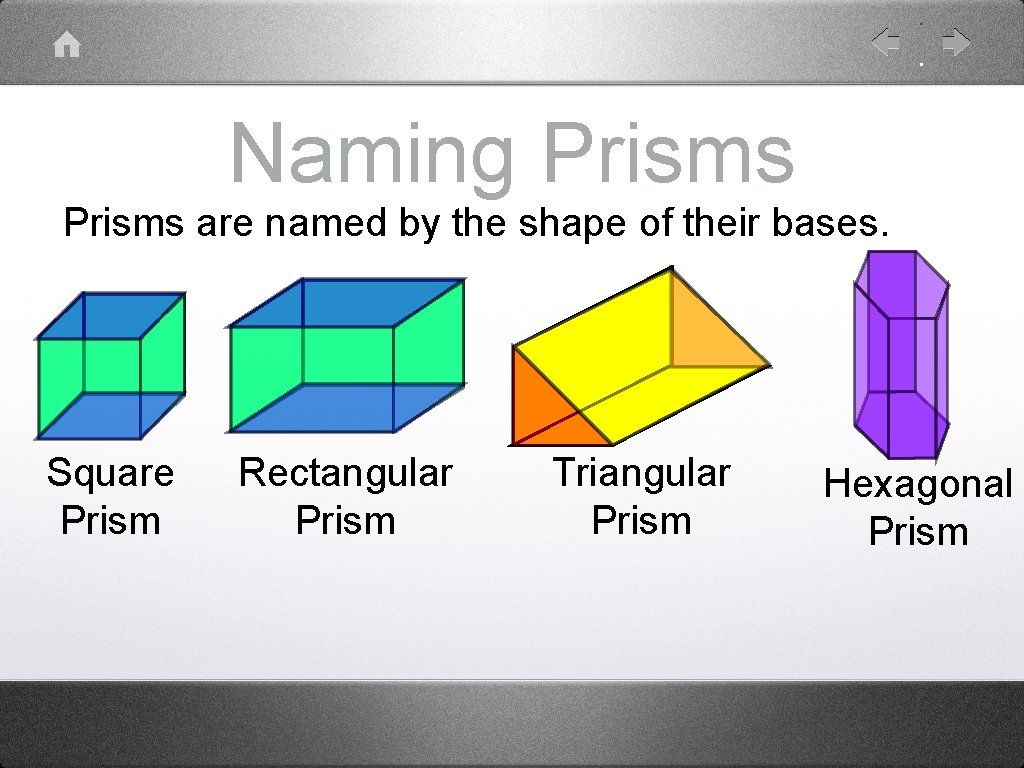Naming Prisms are named by the shape of their bases. Square Prism Rectangular Prism Triangular Prism Hexagonal Prism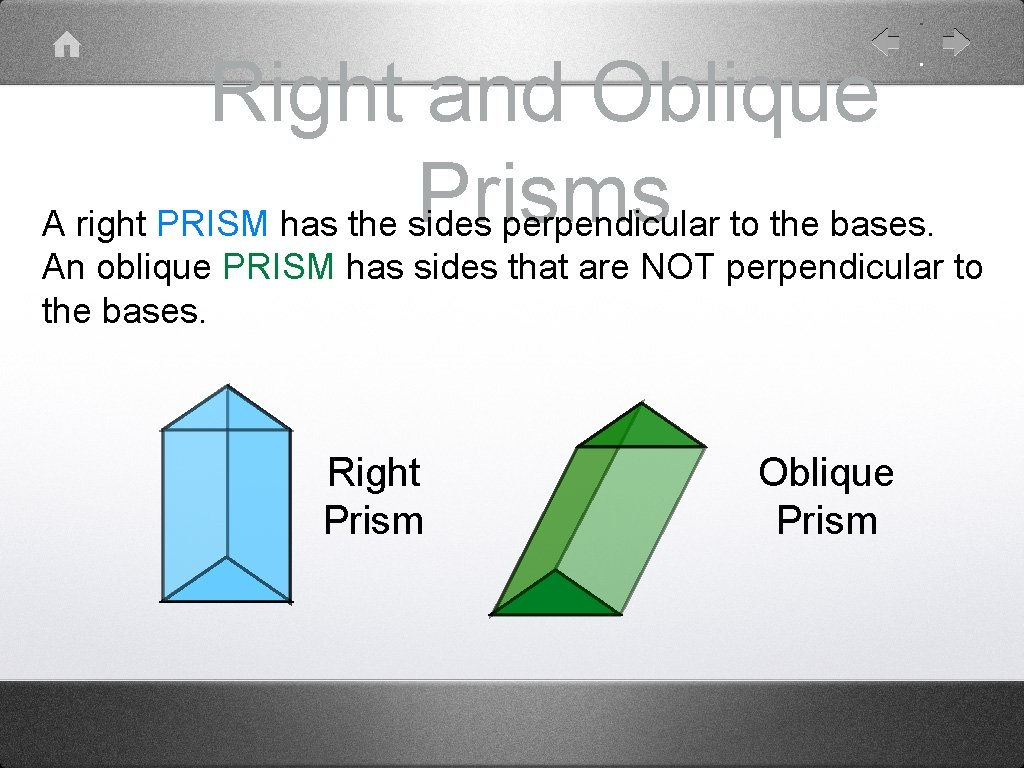Right and Oblique Prisms A right PRISM has the sides perpendicular to the bases. An oblique PRISM has sides that are NOT perpendicular to the bases. Right Prism Oblique Prism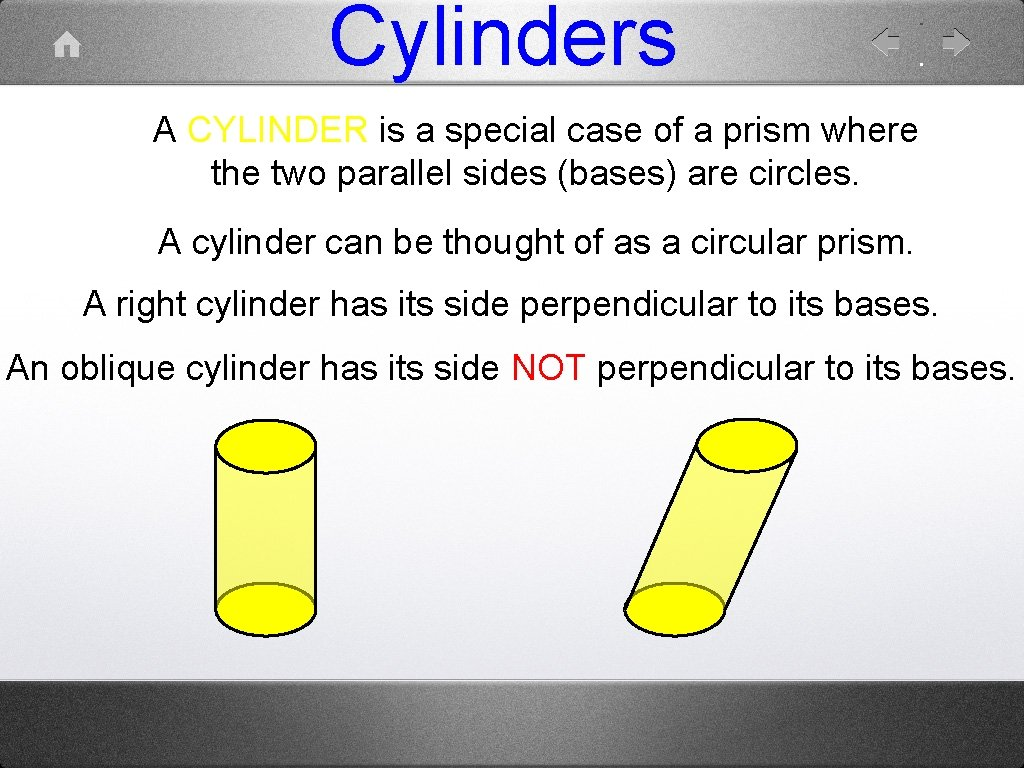Cylinders A CYLINDER is a special case of a prism where the two parallel sides (bases) are circles. A cylinder can be thought of as a circular prism. A right cylinder has its side perpendicular to its bases. An oblique cylinder has its side NOT perpendicular to its bases.Lateral Area of a Lateral Area (LA) is. Prism the area of the side(s) of the object not including the bases. z y z x y x In this case, the LA = (x + y + x + y) z, but x + y + x + y = 2 x + 2 y. This happens to be the perimeter of the base (P). So, the LA of a right prism is given by LA = Ph.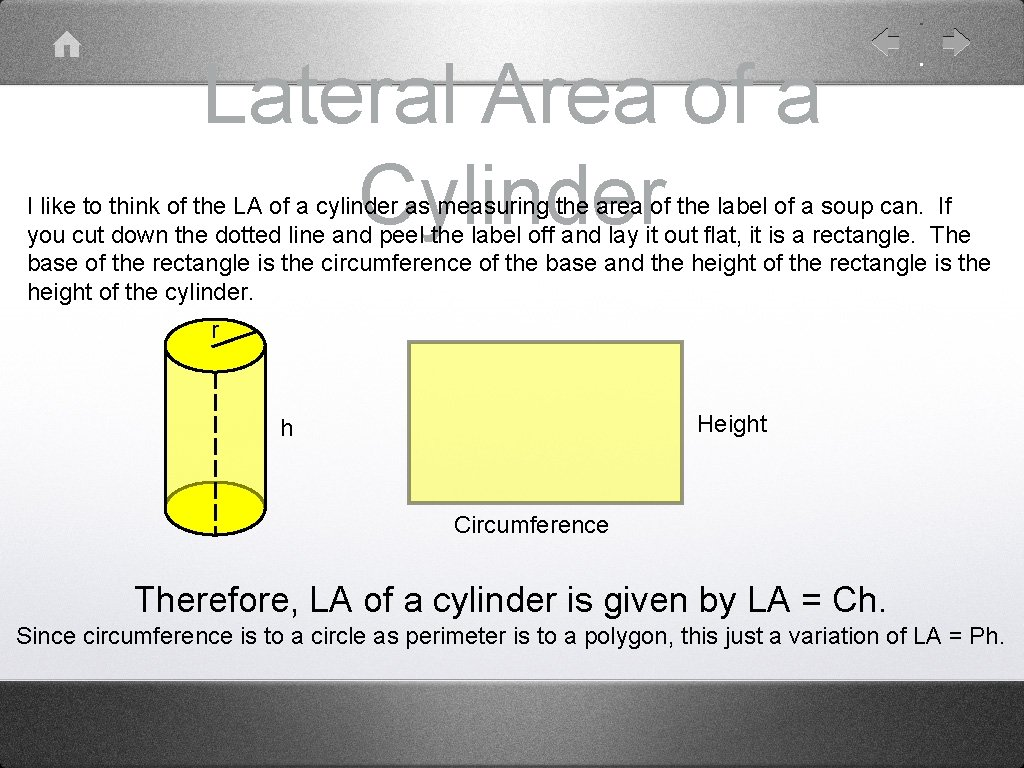Lateral Area of a Cylinder I like to think of the LA of a cylinder as measuring the area of the label of a soup can. If you cut down the dotted line and peel the label off and lay it out flat, it is a rectangle. The base of the rectangle is the circumference of the base and the height of the rectangle is the height of the cylinder. r Height h Circumference Therefore, LA of a cylinder is given by LA = Ch. Since circumference is to a circle as perimeter is to a polygon, this just a variation of LA = Ph.Surface (Total) Area of a Prism The Surface area of a Prism is the sum of the area of ALL of the sides. It is also the LA plus the area of the two bases. Since, however the two bases are congruent, the surface area of a right prism (if B is the area of a base) is given by SA = LA + 2 BExample 1 Find the Surface Area LA = Ph; SA = LA + 2 B LA = Ph; P = 2(12 cm) + 2(10 cm) = 44 cm; h = 8 cm LA = (44 cm)(8 cm) = 352 cm 2 B = (12 cm)(10 cm) = 120 cm 2; so 2 B = 2(120 cm 2) = 240 cm 2 SA = LA + 2 B = 352 cm 2 + 240 cm 2 = 592 cm 2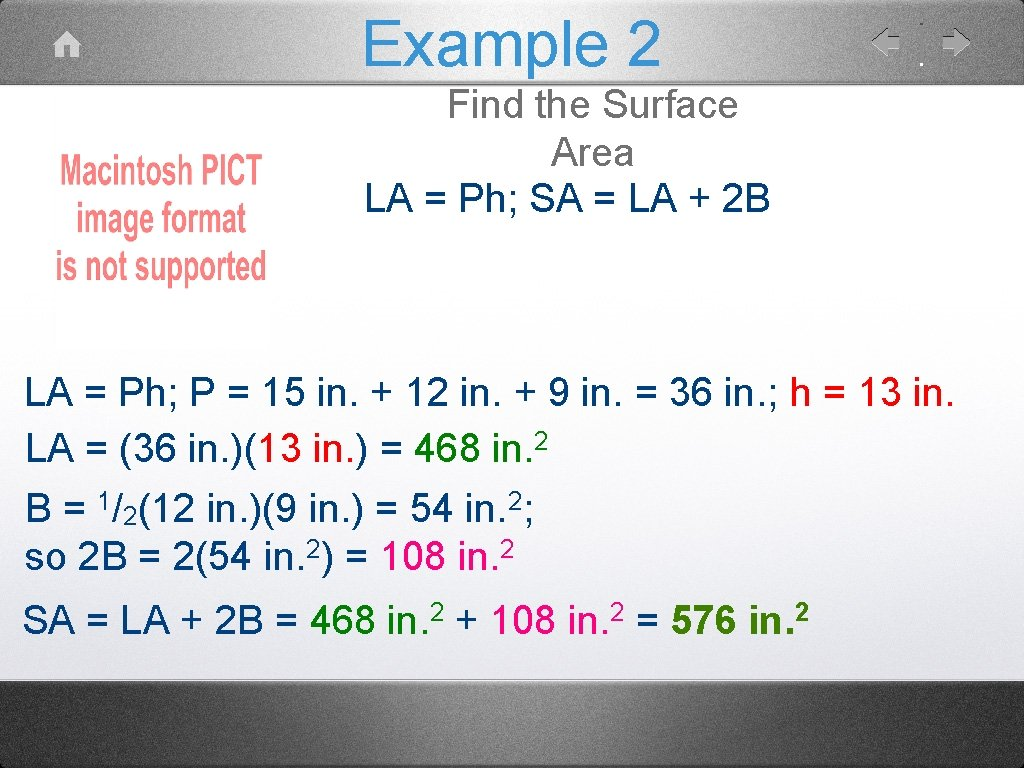Example 2 Find the Surface Area LA = Ph; SA = LA + 2 B LA = Ph; P = 15 in. + 12 in. + 9 in. = 36 in. ; h = 13 in. LA = (36 in. )(13 in. ) = 468 in. 2 B = 1/2(12 in. )(9 in. ) = 54 in. 2; so 2 B = 2(54 in. 2) = 108 in. 2 SA = LA + 2 B = 468 in. 2 + 108 in. 2 = 576 in. 2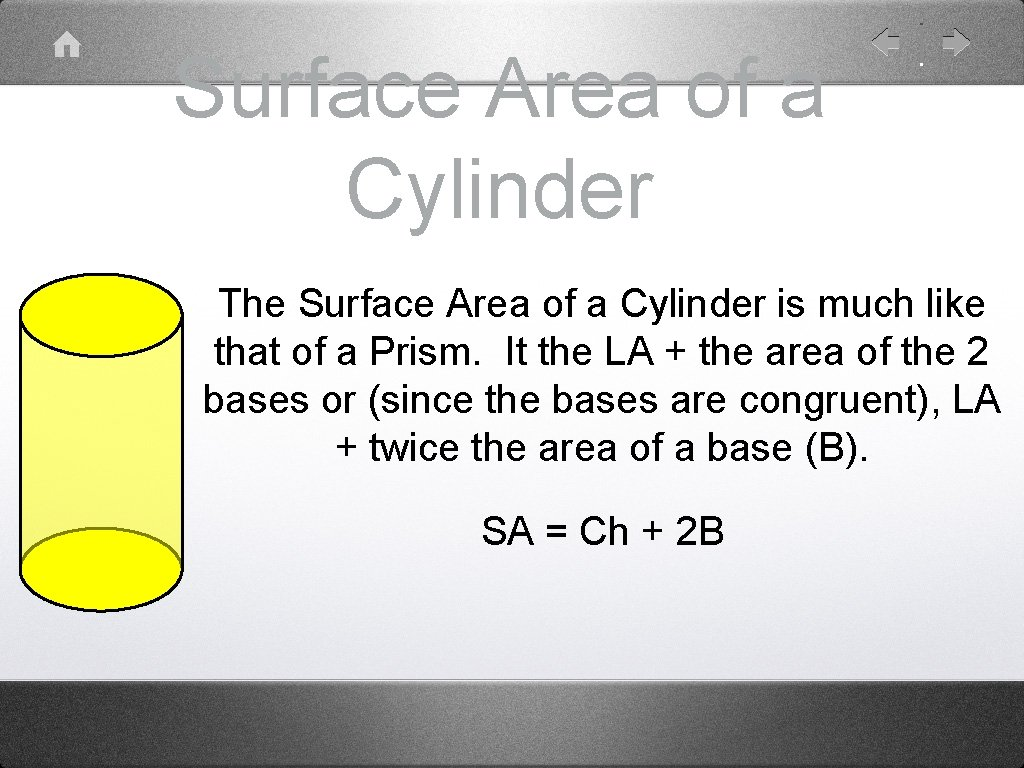Surface Area of a Cylinder The Surface Area of a Cylinder is much like that of a Prism. It the LA + the area of the 2 bases or (since the bases are congruent), LA + twice the area of a base (B). SA = Ch + 2 B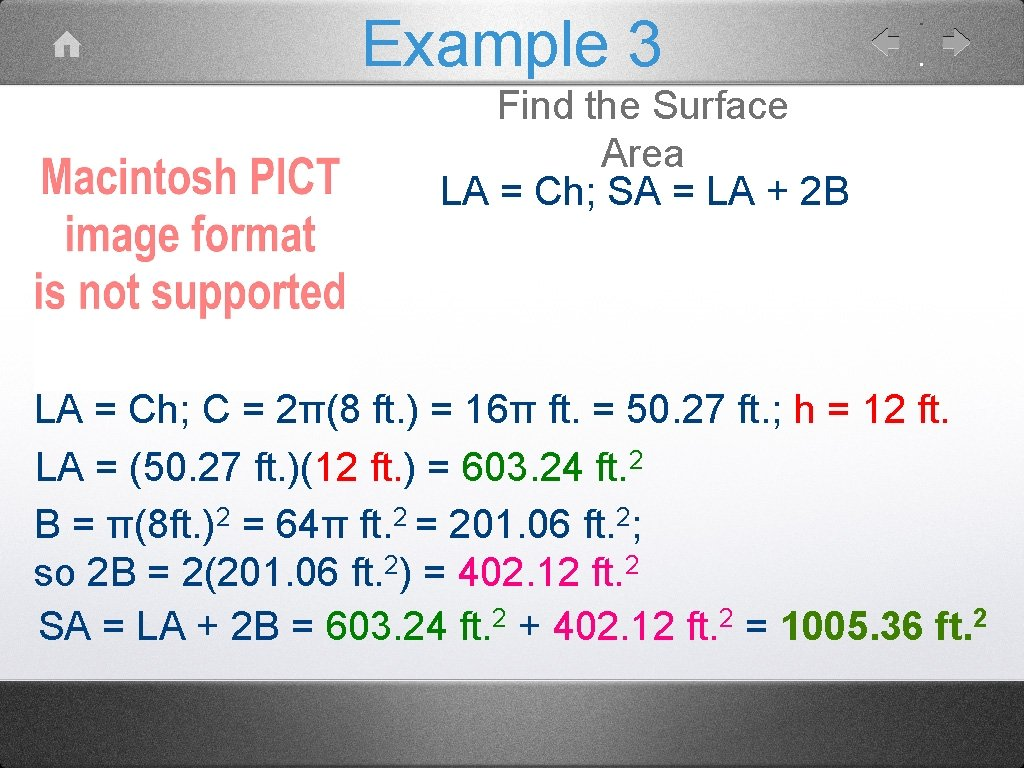Example 3 Find the Surface Area LA = Ch; SA = LA + 2 B LA = Ch; C = 2π(8 ft. ) = 16π ft. = 50. 27 ft. ; h = 12 ft. LA = (50. 27 ft. )(12 ft. ) = 603. 24 ft. 2 B = π(8 ft. )2 = 64π ft. 2 = 201. 06 ft. 2; so 2 B = 2(201. 06 ft. 2) = 402. 12 ft. 2 SA = LA + 2 B = 603. 24 ft. 2 + 402. 12 ft. 2 = 1005. 36 ft. 2Assignment Lateral Area and Surface Area allDog - PRISM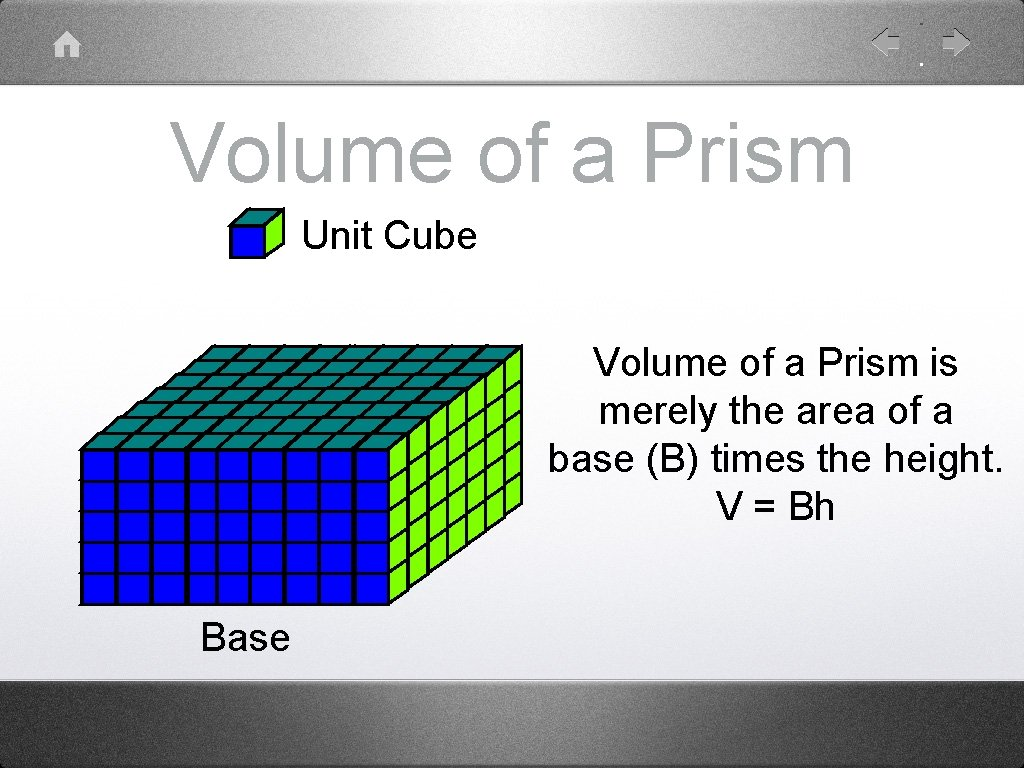Volume of a Prism Unit Cube Volume of a Prism is merely the area of a base (B) times the height. V = Bh Base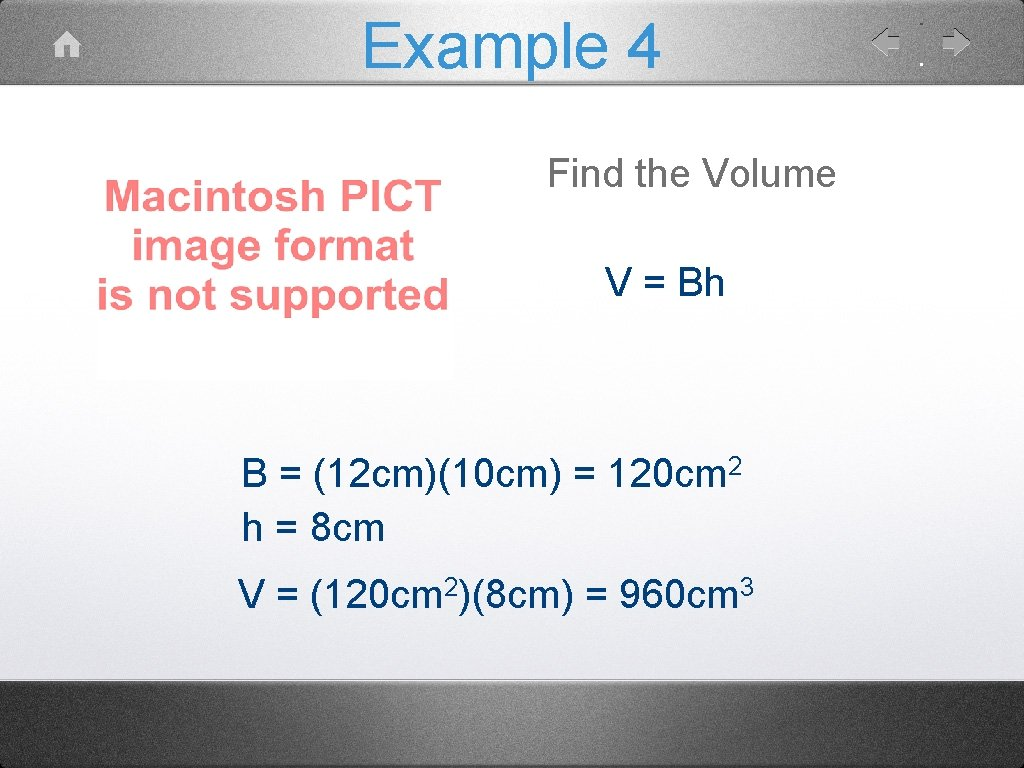Example 4 Find the Volume V = Bh B = (12 cm)(10 cm) = 120 cm 2 h = 8 cm V= 2 (120 cm )(8 cm) = 3 960 cmExample 5 Find the Volume V = Bh B = 1/2(12 in)(9 in) = 54 in 2 h = 13 in. V = (54 in 2)(13 in) = 702 in 3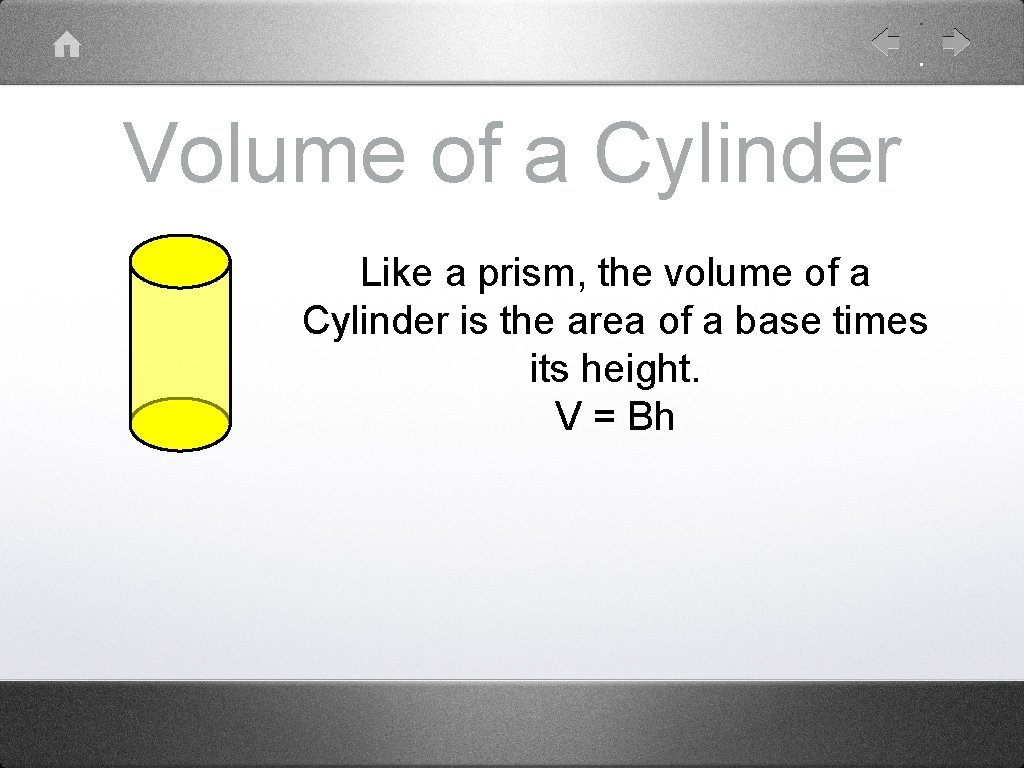Volume of a Cylinder Like a prism, the volume of a Cylinder is the area of a base times its height. V = Bh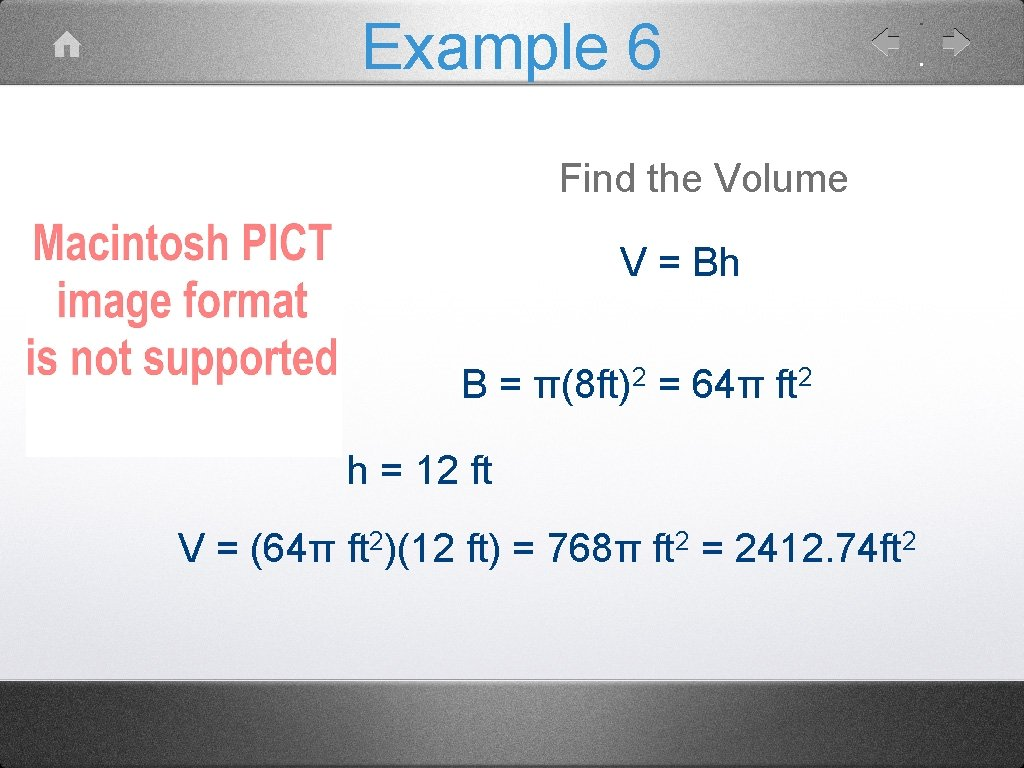Example 6 Find the Volume V = Bh B = π(8 ft)2 = 64π ft 2 h = 12 ft V = (64π ft 2)(12 ft) = 768π ft 2 = 2412. 74 ft 2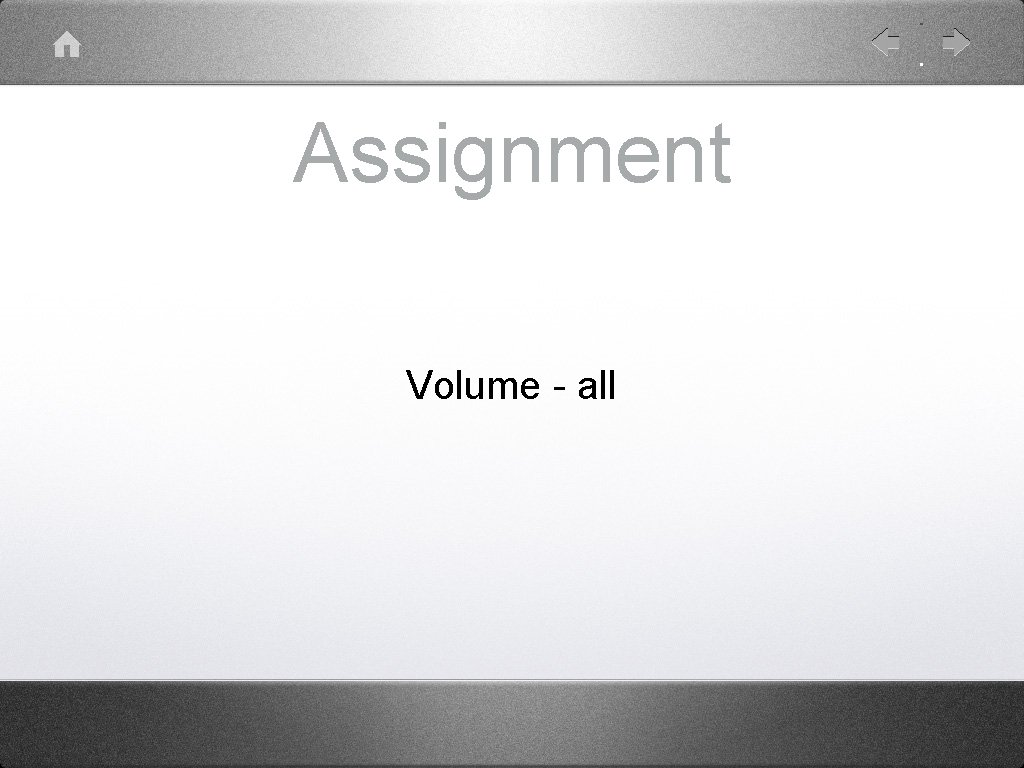Assignment Volume - allPyramids and Cones Square or Triangular Rectangular Cone Pyramid For pyramids and cones V = 1/3 Bh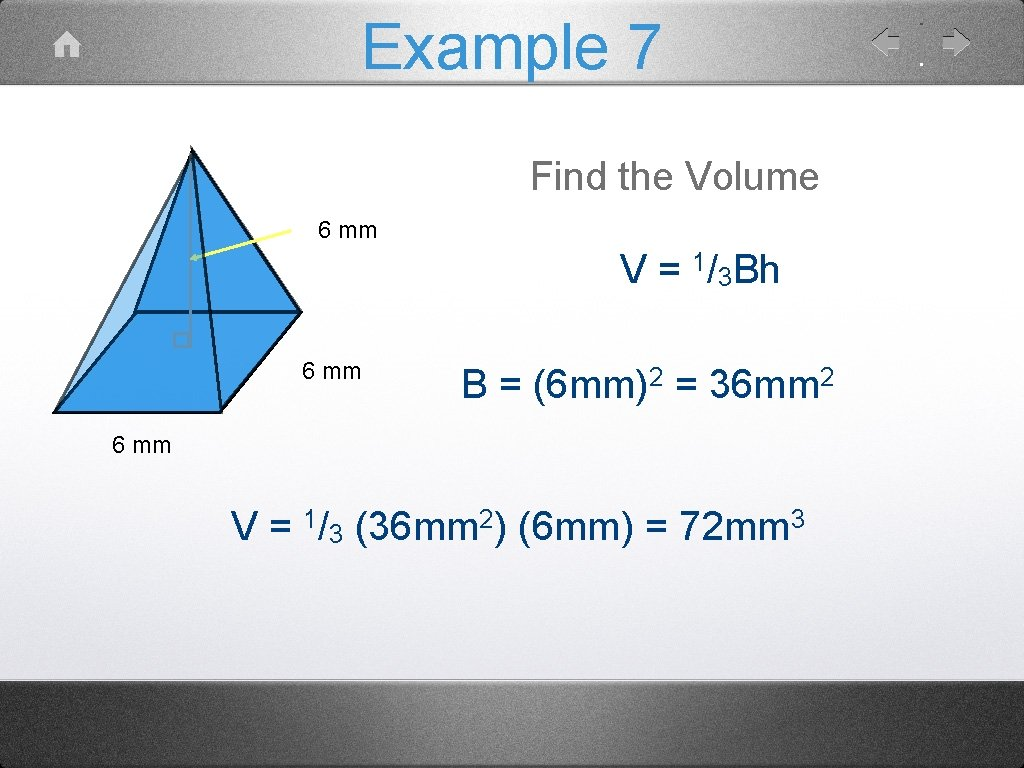Example 7 Find the Volume 6 mm V = 1/3 Bh 6 mm B = (6 mm)2 = 36 mm 2 6 mm V= 1/ 3 2 (36 mm ) (6 mm) = 3 72 mmExample 8 Find the Volume 6 cm V = 1/3 Bh B = 1/2(5 cm)(8 cm) = 20 cm 2 h = 6 cm 8 cm 5 cm V = 1/3 (20 cm 2)(6 cm) = 40 cm 3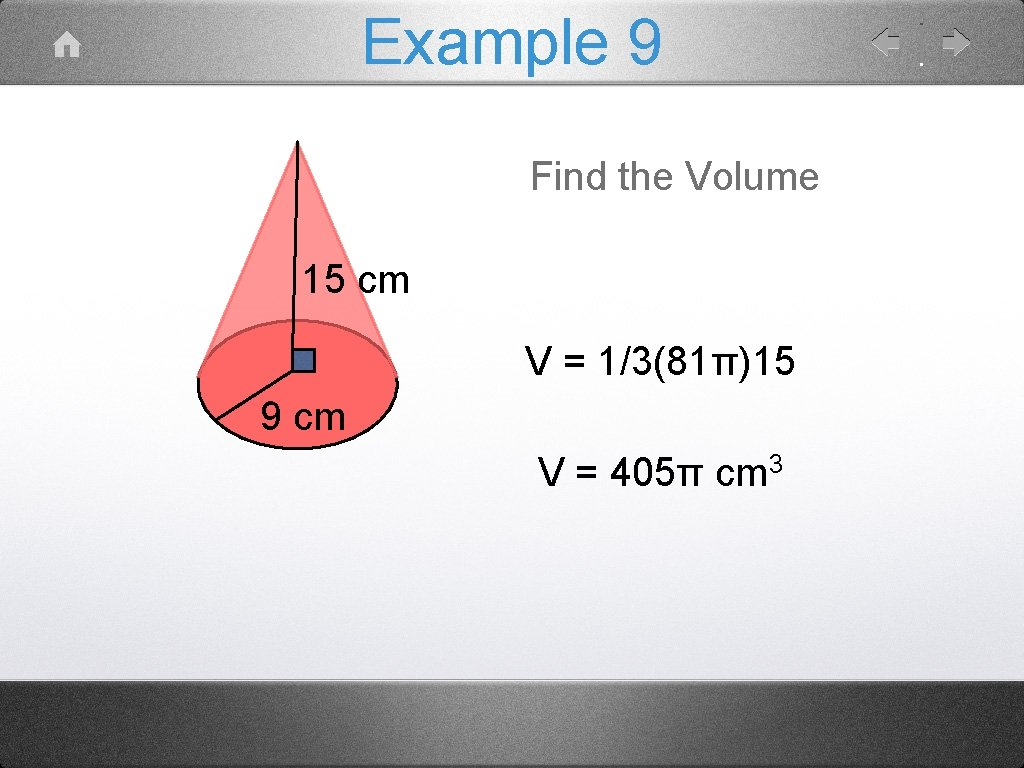Example 9 Find the Volume 15 cm V = 1/3(81π)15 9 cm V = 405π cm 3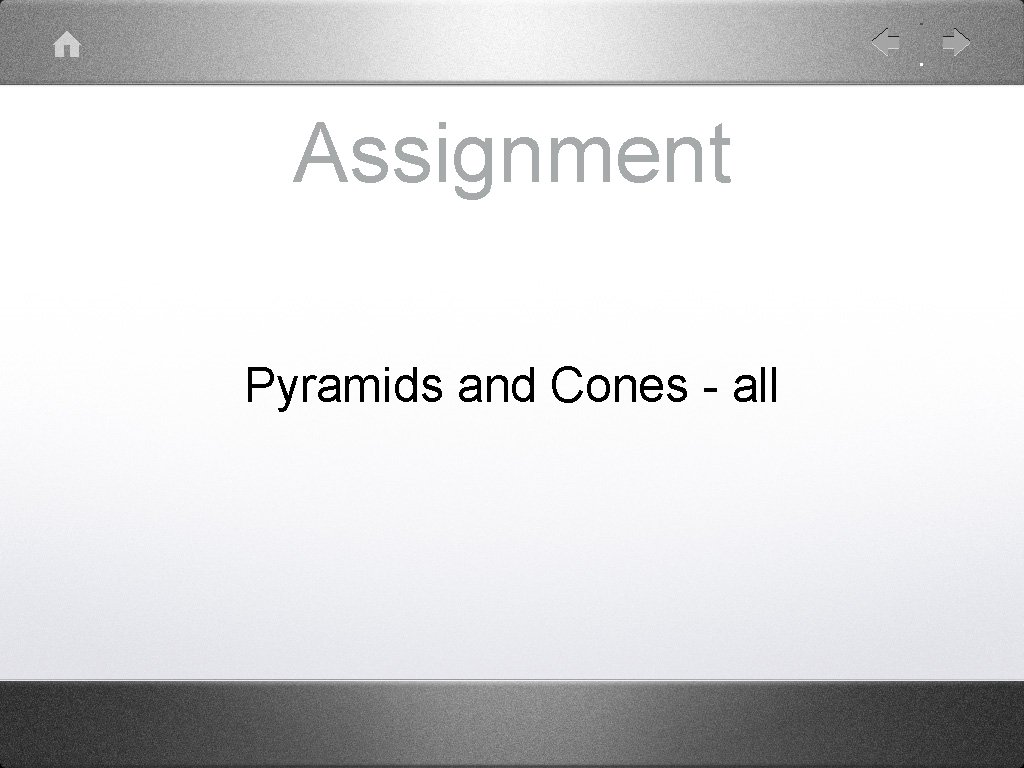Assignment Pyramids and Cones - allSpheres r SA = 4πr 2 V = 4/3πr 3Example 10 Find the Surface Area SA = 4πr 2 7 cm SA = 4π(7 cm)2 SA = 2 196πcm SA = 2 615. 75 cmExample 11 Find the Volume V = 4/3πr 3 12 cm V = 4/3π(12 cm)3 V = 2304πcm 3 V = 7238. 23 cm 3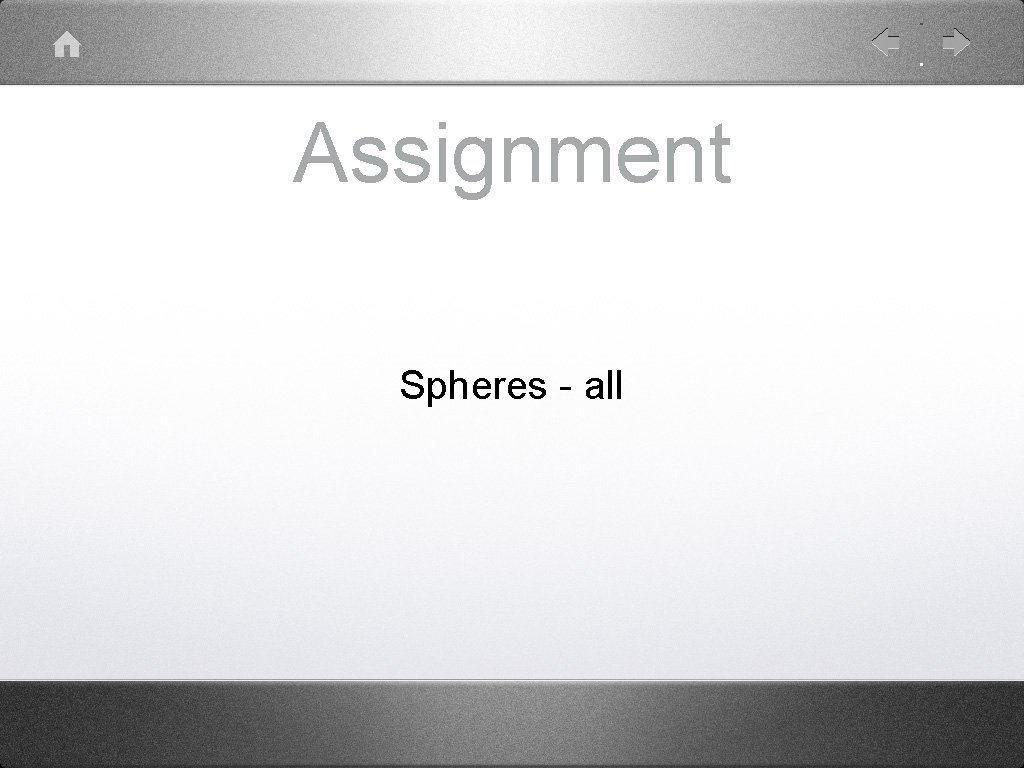Assignment Spheres - all